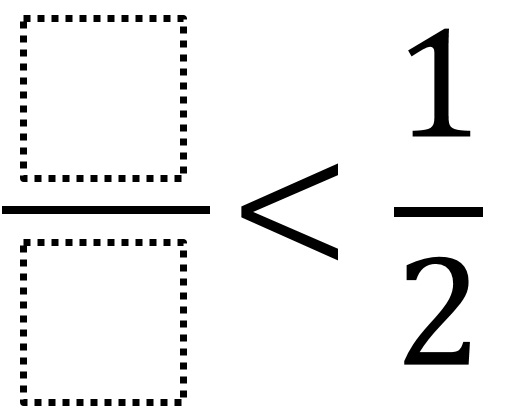Home > Grade 4 > Fractions Less Than One Half

# Fractions Less Than One Half

Directions: Using the digits 1 to 9 at most one time each, fill in the boxes to create as many fractions as possible that are less than one half.### Hint

How can you tell if a fraction is less than one half?
What patterns do you notice?
How can you be certain you have all possible solutions?

16 fractions less than one half
1/9, 2/9, 3/9, 4/9, 1/8, 2/8, 3/8, 1/7, 2/7, 3/7, 1/6, 2/6, 1/5, 2/5, 1/4, 1/3

Source: Christine Newell

## Create A Pattern

Directions: Use the digits 0 to 9, at the most one time each, place a …

1.I’m curious as to why you are counting equivalent fractions separately in your answer. Since 2/8 = 1/4 and 3/9 = 2/6 = 1/3, I initially found only 13 fractions.

•Just seeing this. I would count them as separate answers because although they are equivalent, they have a “different number and size of parts” which is language that comes from a 4th grade standard. A question on the equivalent fractions students find would be a great follow-up question, though!

2.I still wonder what this means lol

3.Christine Newell is right I am a 4th grader and I dont get these so I just wrote them down

4.Your answer uses the digits more than 1 time. Are they just meant to be possibilities?